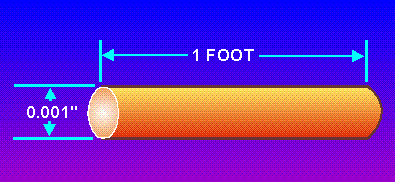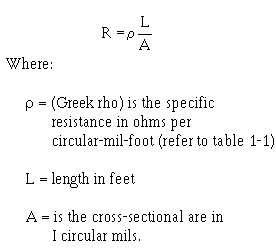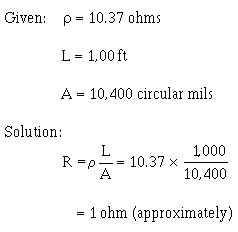Circular Mil-FootCustom SearchCIRCULAR-MIL-FOOT

A circular-mil-foot (figure 1-3) is a unit of volume. It is a unit conductor 1 foot in length and has a cross-sectional area of 1 circular mil. Because it is a unit conductor, the circular-mil-foot is useful in making comparisons between wires consisting of different metals.

For example, a basis of comparison of the RESISTIVITY (to be discussed shortly) of various substances may be made by determining the resistance of a circular-mil-foot of each of the substances.

Figure 1-3. - Circular-mil-foot.In working with square or rectangular conductors, such as ammeter shunts and bus bars, you may sometimes find it more convenient to use a different unit volume. A bus bar is a heavy copper strap or bar used to connect several circuits together. Bus bars are used when a large current capacity is required.

Unit volume may be measured as the centimeter cube. Specific resistance, therefore, becomes the resistance offered by a cube-shaped conductor 1 centimeter in length and 1 square centimeter in cross-sectional area. The unit of volume to be used is given in tables of specific resistances.

SPECIFIC RESISTANCE OR RESISTIVITY

Specific resistance, or resistivity, is the resistance in ohms offered by a unit volume (the circular-mil-foot or the centimeter cube) of a substance to the flow of electric current. Resistivity is the reciprocal of conductivity.

A substance that has a high resistivity will have a low conductivity, and vice versa. Thus, the specific resistance of a substance is the resistance of a unit volume of that substance.

Many tables of specific resistance are based on the resistance in ohms of a volume of a substance 1 foot in length and 1 circular mil in cross-sectional area. The temperature at which the resistance measurement is made is also specified. If you know the kind of metal a conductor is made of, you can obtain the specific resistance of the metal from a table. The specific resistances of some common substances are given in table 1-1.

Table 1 - 1. - Specific Resistances of Common Substances

 Substance Specific resistance at 20°C. Centimeter cube (microhms) Circular-mil-foot (ohms) Silver 1.629 9.8 Copper (drawn) 1.724 10.37 Gold 2.44 14.7 Aluminum 2.828 17.02 Carbon (amorphous) 3.8 to 4.1 Tungsten 5.51 33.2 Brass 7.0 42.1 Steel (soft) 15.9 95.8 Nichrome 109.0 660.0

The resistance of a conductor of a uniform cross section varies directly as the product of the length and the specific resistance of the conductor, and inversely as the cross-sectional area of the conductor. Therefore, you can calculate the resistance of a conductor if you know the length, cross-sectional area, and specific resistance of the substance. Expressed as an equation, the "R" (resistance in ohms) of a conductor isProblem:

What is the resistance of 1,000 feet of copper wire having a cross-sectional area of 10,400 circular mils (No. 10 wire) at a temperature of 20°C?

Solution:

The specific resistance of copper (table 1-1) is 10.37 ohms. Substituting the known values in the preceding equation, the resistance, R, is determined asIf R, &rho;, and A are known, the length (L) can be determined by a simple mathematical transposition. This has many valuable applications. For example, when locating a ground in a telephone line, you will use special test equipment.

This equipment operates on the principle that the resistance of a line varies directly with its length. Thus, the distance between the test point and a fault can be computed accurately.

Q.7 Define specific resistance.Q.8 List the three factors used to calculate resistance of a particular conductor in ohms.Integrated Publishing, Inc. - A (SDVOSB) Service Disabled Veteran Owned Small Business